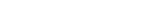MathExamle.com# Systems of Equations

Create worksheets with systems of equations, select type of a generator and viewing some samples of task

Creates system of equations, the answers are always integers and positive

3 Variants 3 Levels
 13x÷y=13 y×3x=300
 6x×4y=960 132y÷10x=33
Variant: A Level: Easy

Creates system of equations, the answers are integers and can be negative

3 Variants 3 Levels
 -3y×12x=-324 30x÷6y=5
 2x×y=160 336y÷2x=210
Variant: A Level: Easy